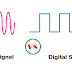The actual difference between Analog and Digital Signal is, an Analog signal is a continuous signal which gives different values over time, whereas the digital signal is a discontinuous or discrete signal which gives only two values over time either Zero(0) or One(1).
Here you will find a lot of important differences between Digital and Analog Signal with proper examples.

## Differences between Analog and Digital Signal

1. Analog Signal is a continuous signal whereas the digital signal is a discontinuous or discrete signal.

2. Analog signal carries so many different values over time but the digital signal carries only two values either High(1) or Low(0).

3. Analog signals can carry more information than digital signals whereas digital signals can carry less information than analog signals.

4. Analog signals can carry more accurate information but digital signals can carry less accurate information.

5. Analog signals cannot be stored easily because they can carry infinite values, so you need infinite memory to store analog signals. On the other hand, digital signals can be stored easily.

6. As the analog signals carry a huge number of values so they are difficult to analyze whereas digital signals can be analyzed very easily.

7. Analog signals are denoted by Sine waves which indicates a continuous signal which changing over time. Digital signals are denoted by square waves which indicates a discontinuous or discrete signal.

8. Analog signals carry so much noise, sometimes noise cannot be fully removed from an analog signal. Digital signals carry almost no noise if they carry noise we can remove easily.

9. More loss occurs when analog signals are transmitted, whereas less loss occurs when digital signals are transmitted.

10. Analog signals most suitable for wireless data transmission, almost all wireless data transmission uses analog signals. Digital signal not so suitable for wireless data transmission.

11. Analog signals are described by amplitude, phase, and frequency whereas digital signals are described by bit rate, bit intervals, duty cycles, etc.

12. Analog signal has no fixed its amplitude may be reached up to infinite but digital signal has a fixed range. Zero(0) to five(5) volt generally used for digital signals.

13. Digital signals can be transmitted with a very low transmission cost where analog transmission required more cost.

14. Analog signals are most suitable for audio transmission whereas digital signals are most suitable for image, video, etc transmission.

## Analog Signal Examples

The best example of Analog signal is human voice or any other sound. Other examples of analog signals are hydraulic, mechanical instruments, air pressure meters, etc. Almost all transducers can create only analog signals.

## Digital Signal Examples

The best example of digital signal is our smartphone camera. When we capture any photo then digital signals are generated. Other examples of digital signals are computer keyboards, digital scanner, printers, etc. They all are communicated through digital signals.

[Actual] Difference between Analog and Digital Signal with ExamplesReviewed by manoranjan das on 10:40 pm Rating: 5